Next: The AUSTRALIAN data set Up: Classification with mixtures of Previous: Classification with mixtures of

Using a mixture of trees as a classifier

A density estimator can be turned into a classifier in two ways, both of them being essentially likelihood ratio methods. Denote the class variable by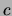and the set of input variables by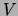. In the first method, adopted in our classification experiments under the name of MT classifier, an MT model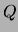is trained on the domain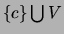, treating the class variable like any other variable and pooling all the training data together. In the testing phase, a new instance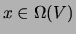is classified by picking the most likely value of the class variable given the settings of the other variables: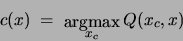Similarly, for the MF classifier (termed D-SIDE'' by [Kontkanen, Myllymaki, Tirri 1996]),above is an MF trained on. The second method calls for partitioning the training set according to the values of the class variable and for training a tree density estimator on each partition. This is equivalent to training a mixture of trees with observed choice variable, the choice variable being the class[Chow, Liu 1968,Friedman, Geiger, Goldszmidt 1997]. In particular, if the trees are forced to have the same structure we obtain the Tree Augmented Naive Bayes (TANB) classifier of [Friedman, Geiger, Goldszmidt 1997]. In either case one turns to Bayes formula: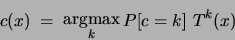to classify a new instance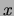. The analog of the MF classifier in this setting is the naive Bayes classifier.Next: The AUSTRALIAN data set Up: Classification with mixtures of Previous: Classification with mixtures of
Journal of Machine Learning Research 2000-10-19